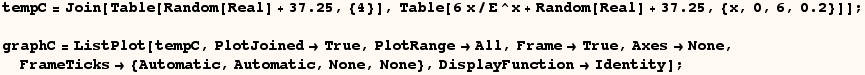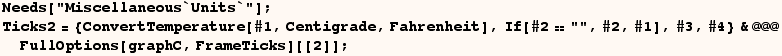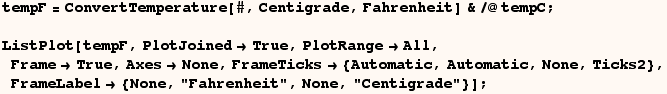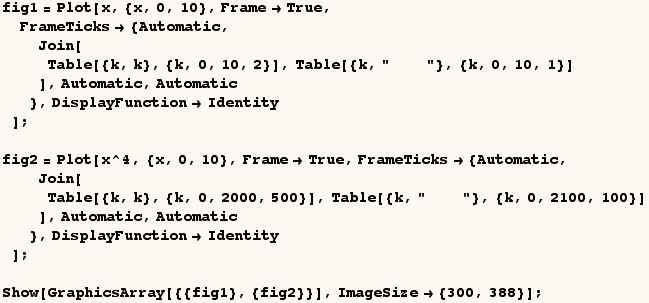FrameTicks

A user wanted to present data on the y axis and refer to it with 2 equivalent scales
(degrees Fahrenheit and degrees Celcius)

Wolf Hartmut gave a solution where he demonstrates by first defining a fever curve (temp) in Centigrade units, and a Plot of the curve is assigned to graphC.Ticks2 are the original FrameTicks redefined so the text for Clesius degrees are placed at the equivalent place on a graph with Farenheight units.Next the fever curve is converted to Fahrenheit, and  this is plotted with Ticks2.  The altered FrameTicks are used on the left edge of the graph.A user wanted to have two graphs displayed one above the other in a GraphicsArray, and
have the vertical axes alligned with eachother

Bob Hanlon recommended using an identical but  "invisible" FrameTick on each graph's axis that is slightly wider than the widest actual tick label as below.Created by Mathematica  (May 16, 2004)

Back to Ted’s Tricks index page